# JEE Main 2020 Question Paper with Solution (Jan 7th second shift)

## 7th January, Afternoon Session ( 2:30 pm to 5:30 pm)

National Test Agency( NTA) Is conducting JEE Mains 2020 Exam in two shifts, through an online mode. JEE Main 2020 is a computer-based test. In this article, we are going to share a JEE main 2020 question paper with solution for the afternoon session of 7th January 2020. These solutions are prepared by experts. We suggest you go through the article once, it will help you to understand your performance and with the help of this article, you will be able to understand that which concept is important, Type of exam questions, etc.

Exam date and Shift Article URL

## JEE Main 2020 exam - Chemistry ( 7th January second shift)

Q1. The number of sp2 hybridized carbon atoms in aspartame.

Solution

The aspartame structure is given below: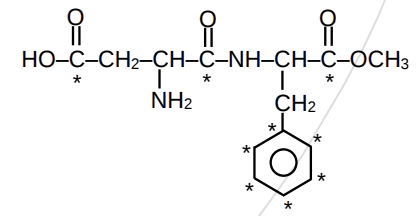The number of sp2 hybridised carbon atoms in aspartame is 9.

Q2.  Find the bond order and magnetic nature of CN-.

Solution

The bond order of CN- is 3 and all the electrons are paired in this ion, thus it is diamagnetic in nature.

Q3.  Which one of the two elements among the following pairs (F, Cl), (S, Se) and (Li, Na) will release more energy on gaining of an electron?

Solution

From the given pairs, the more energy on gaining of an electron will be released by the following elements:
Cl, S, and Li

Q4. 3 grams of acetic acid is mixed in 250 mL of 0.1 M HCl. This mixture is now diluted to 500 mL. 20 mL of this solution is now taken in another container and 1/2 mL of 5M NaOH is added to this. Find the pH of this solution.
(log 3 = 0.4771, pKa = 4.74)

Solution
m mole of acetic acid in 20 mL = 2
m mole of HCl in 20 mL = 1
m mole of NaOH = 2.5

2                              3/2                                      0                        0
0.5                             0                                       3/2

pH = 4.74 + log 3

pH = 4.74 + 0.48 = 5.22

Thus, the correct answer is 5.22

Q5. The standard enthalpy of formation(in kJ/mol) of ethane, it the enthalpies of combustion of ethane, dihydrogen, and C(graphite) are -1560kJ/mol, -286kJ/mol and -393.5kJ/mol respectively is:

Solution

The reactions we have:

...................(i)

..........................(ii)

........................(iii)

Multiply eq(ii) by 2 and eq(iii) by 3.

Now, Hof = 2 x eq(ii) + 3eq(iii) - 1

Thus, the equaton is given as:

Q6.Select the correct options :
(1) A = CMPS, B = CAverage, C = CRMS,                                     (2) A = CAverage, B = CMPS, C = CRMS
(3) A = CRMS, B = CAverage, C = CMPS,
(4) A = CAverage, B = CMPS, C = CRMS

Solution

CRMS > CAverage > CMPS.

Opiton 1 is correct.

Q7.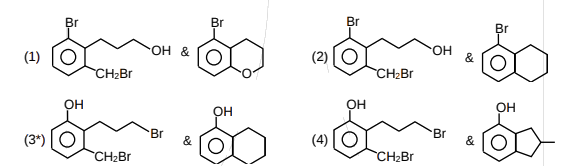SolutionOption 3 is correct.

Q8. A and B are in the given reaction ?SolutionOption 1 is correct.

Q9. Amongs the following which is a redox reaction?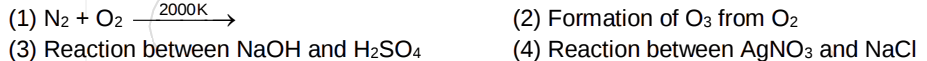SolutionOption 1 is correct.

Q10.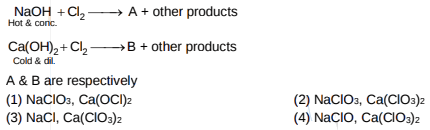Solution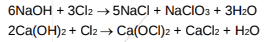Option 1 is correct.

Q11.  There are two beakers  (I) having a pure volatile solvent and (II) having a volatile solvent and non-volatile solute. If both beakers are placed together in a closed container then:

(1) Volume of solvent beaker will decrease and solution beaker will increase

(2) Volume of solvent beaker will increase and solution beaker will also increase

(3) Volume of solvent beaker will decrease and solution beaker will also decrease

(4) Volume of solvent beaker will increase and solution beaker will decrease

Solution

There will be lowering in vapour pressure in the second beaker.

Option 1 is correct.

Q12.   Metal with low melting point containing impurities of high melting point can be purified by
(1) Zone refining                         (2) Vapor phase refining
(3) Distillation                              (4) Liquation

Solution

Concept-based.

Option 4 s correct.

Q13.  Which of the following statements are correct?
(I) On the decomposition of H2O2, O2 gas is released .
(II) 2-ethylanthraquinol is used in preparation of H2O2
(III) On heating KClO3, Pb(NO3)2, NaNO3, O2 gas is released.
(IV) In the preparation of sodium peroxoborate, H2O2 is treated with sodium metaborate.

(1) I, II, IV               (2) II, III, IV                (3) I, II, III, IV                (4) I, II, III

Solution

Concept based.

Option 3 is correct.

Q14. Which of the following reactions are possible?Solution

1. Vinyl halides (C)  do not give Fridel Craft's reaction becuase of unstable carbocation formation.

2. Aryl halides (A) do not give Fridel Craft's reaction with Benzene because of formation of unstable phenyl carbocation.

Option 2 is correct.

Q15. The correct statement about gluconic acid is

(1) It is prepared by oxidation of glucose with HNO3

(2) It is obtained by partial oxidation of glucose

(3) It is a dicarboxylic acid

(4) It forms hemiacetal or acetal

Solution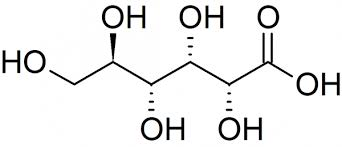Gluconic acid is obtained by partial oxidation of glucose by Tollen's reagent or Fehling solution or Br2,H2O. Gluconic acid can not form hemiacetal or acetal.

option 2 is correct.

Q16. The standard enthalpy of formation(in kJ/mol) of ethane, it the enthalpies of combustion of ethane, dihydrogen, and C(graphite) are -1560kJ/mol, -286kJ/mol and -393.5kJ/mol respectively is:

Solution
The reactions we have:

...................(i)

..........................(ii)

........................(iii)

Multiply eq(ii) by 2 and eq(iii) by 3.

Now, Hof = 2 x eq(ii) + 3eq(iii) - 1

Thus, the equaton is given as:

Q17.  Flocculation value for As2S3 sol by HCl is 30 mmoleL–1 . Calculate mass of H2SO4 required in gram for 250 mL sol.

Solution

For 1L sol 30 mmol of HCl is required.

Thus, for 1L sol 15 mmol H2SO4 is required

For 250 mL of sol:
(15/4) x 10-3 mmol H2SO4 = 0.3675 g

Thus, the correct answer is 0.37

Q18.

Determine total number of atoms per unit formula of (A), (B) and (C).

Solution

(A) = CrO2Cl2

(B) = Na2CrO4

(C) = CrO5

Thus, the correct answer is 18.

Q19. 0.6 g of urea on strong heating with NaOH evolves NH3. Liberated NH3 will combine completely with which of the following HCl solution?

(1) 100 mL of 0.2 N HCl

(2) 400 mL of 0.2 N HCl

(3) 100 mL of 0.1 N HCl

(4) 200 mL of 0.2 N HCl

Solution

2 × mole of Urea = mole of NH3 ........(1)
mole of NH3 = mole of HCl ........(2)
mole of HCl = 2 × mole of Urea = 2 x (0.6/60) = 0.02 mol

100 x 0.2 x 1= 0.02 mol,  from 1.

So, option 1 is correct.

Q20. Which of the following statements are incorrect?

(A) Co+3 with strong field ligand forms a high magnetic moment complex.

(B) For Co+3 if pairing energy(P) >  then the complex formed will have  a configuration

(C) For [Co(en)3] 3+  is less than for [CoF6] 3–

(D) If  = 18000 cm–1 for Co+3 then with same ligands for it  = 16000 cm–1

(1) A, D

(2) B, C

(3) A, B

(4) A, B, C, D

Solution

Concept-Based.

Option 1 is correct.

Q21. Stability order of following alkoxide ions is.Solution

When negative charge is delocalised with electron withdrawing group like (NO2) then stability increases.

(A) Negative charge is localised

(B) Negative charge is delocalised with NO2 group

(C) Negative charge is delocalised with carbon of alkene

Option 1 is correct.

Q22. For the complex [Ma2b2] if M is sp3 or dsp2 hybridised respectively then total number of optical isomers are respectively :

(1) 1, 1                         (2) 2, 1                          (3) 0, 0                         (4) 1, 2

Solution

Both will not show optical isomerism.

Option 3 is correct.

## JEE Main 2020 exam - Mathematics ( 7th January second shift)

Q.1  if the sum of the first 40 terms of the series 3+4+8+9+13+14+18+19+...... Is 102m then what is the value of m

Solution

Q2.  What is the coefficient of  in the expansion of  is?

Solution

Q3.  Find the area between the curve

Solution

Point of intersection (-1,4),(3,36)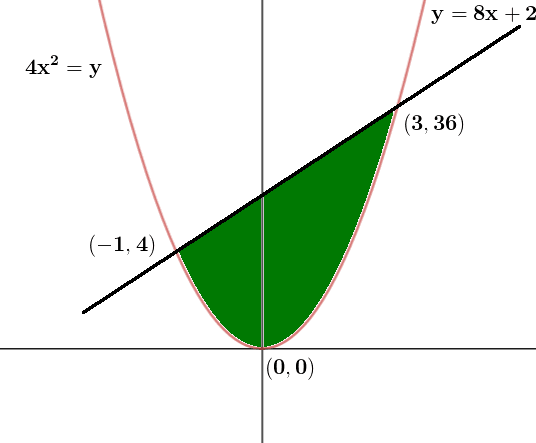Q4. Let A = [aij], B=[bij] are two 3 × 3 matrices such that  & |B| = 81. Find |A|  if  = 3.

Solution:

Taking Common  from  and  from

Taking Common  from  and  from

Q5. Pair of tangents are drawn from origin to the circle then the square of the length of the chord of contact is?

Solution

Length of the tangent from the origin (0,0) is

Length of Chord of contact

Q6. The contrapositive of if  and  then

Solution:

Contrapositive of

Q7.  Let y(x) is the solution of the differential equation ,  then find the value of x where curve cuts the x-axis

Solution:

Hence

Q8.   are two solutions of  then  equal to

Solution:

Q9.  If  , then a number of ordered pairs (r, k) are –(where ).

Solution

r should be less than or equal to 35

Hence r can be 5 and 35

For r=5 we get k=-2,-5

For r=35 we get k=-3,3

We get 4 ordered pair

Q10.

Solution

Q11.  Let f(x) is a five-degree polynomial that has critical points  and  then which one is incorrect.

(1) f(x) has minima at x = 1 & maxima at x = –1
(2) f(1) –4f(–1) = 4
(3) f(x) is maxima at x = 1 and minima at x = –1
(4) f(x) is odd

Solution

On solving

f(x) is maxima at x = 1 and minima at x = –1

Q12.  If  are unit vectors such that and and  and  then

Solution

Q13. Let  if LMVT is applicable on  the value of c is :

Solution

f(x) is a polynomial function
Therefore, it is continuous and differentiable in [0, 1]
Here f(0) = 11, f(1) = 1 – 4 + 8 + 11 = 16

Q14. There are 5 machines. The probability of a machine being faulted is . Probability of atmost two machines is faulted, is then the value of k is

Solution

Required probability = when no. the machine has fault + when only one machine has fault + when only two machines have the fault.

Q15.  Let  and  are the roots of such that  then which one is incorrect?

Solution

Q16. From any point P on line x = 2y perpendicular is drawn on y = x. Let foot of perpendicular is Q. Find
the locus of midpoint of PQ.

Solution

Using midpoint

so locus is

Q17.  If mean and variance of 2, 3, 16, 20, 13, 7, x, y are 10 and 25 respectively then find xy ?

Solution

Q18. If the system of equation x + y + z = 6,  x + 2y + 3z = 10,  3x + 2y + z =  has more than two solutions, then value of

Solution

x + y + z = 6 …….. (1)

x + 2y + 3z = 10 …….. (2)

3x + 2y + z = …….. (3)

from (1) and (2)

if z = 0  x + y = 6 and  x + 2y = 10

y = 4, x = 2

(2, 4, 0)

if y = 0  x + z = 6 and  x + 3z = 10

z = 2 and x = 4

(4, 0, 2)

so, 3x + 2y + z =  must pass through  (2, 4, 0) and  (4, 0, 2)

= 14

and 12 + 2 =

= 1

= 13

Q19.  If f(x) is defined in

Find k such that f(x) is continuous

Solution

Q20. If  is foot of perpendicular drawn from P(1, 0, 3) on a line L and if line L is passing through (, 7, 1), then value of is

Solution

Since PQ is perpendicular to L, therefore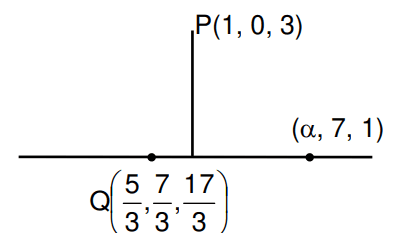Q21.

Then find number of elements in the smallest subset of X which contain elements of both A and B

Solution

Q22.

If

Solution

Q23.  a1, a2, a3 …..a9 are in GP where a1 < 0, a1 + a2 = 4, a3 + a4 = 16, if

then find the

Solution

a1 + a2 = 4 a1 + a1r = 4 ………(i)

a3 + a4 = 16  a1r2 + a1r3 = 16 …….(ii)

Q24.

Solution

as  z is purely real

Q25.   3x + 4y = 12 is the tangent to the ellipse  then the distance between focii of ellipse is-

Solution

condition of tangency

distance between foci =

JEE Main 2020 exam - Physics ( 7th January second shift)

Q1.  What is the dimensional formula of , where B is the magnetic field and  is permeability of free space?

Solution

The energy density of Electromagnetic wave=

So,

Q2.  Moment of inertia of a colid disc as mass per unit area varies as A+Bx2, given the radius of the body is 'a':-

solution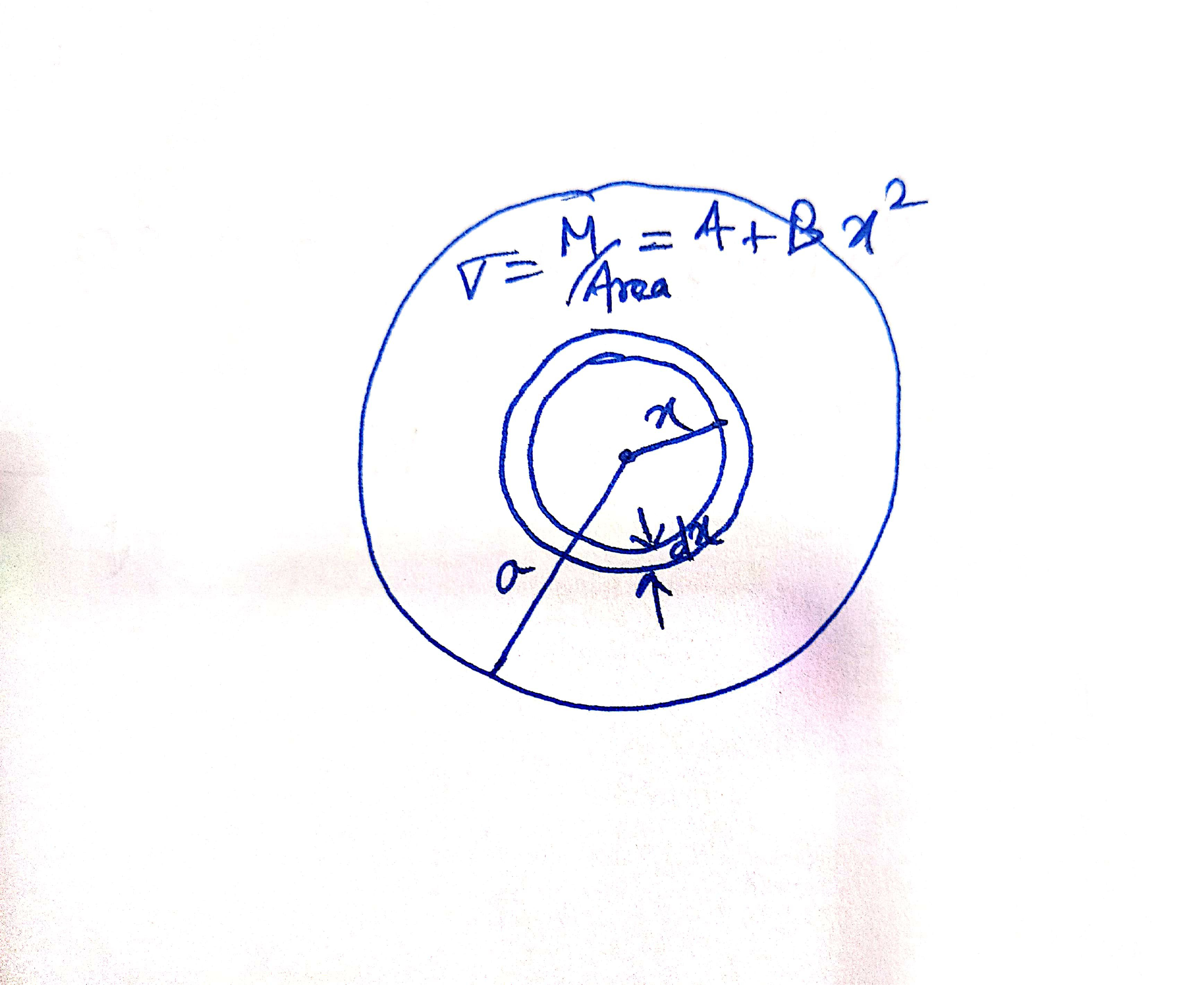We take an elemental ring of mass dm at a distance x from the centre.

So Area of an elemental ring=

As moment of inertia for an elemental ring =

Total  moment of inertia=

Q3.  A lift can hold a maximum of 10 people, each of mass 68 kg, a mass of lift is 920 kg, friction of 6000 N is acting on it. Lift is moving with a velocity of 3 m/s. If a lift is moving upward, calculate the power supplied by the motor to the lift, it is moving with its maximum intensity.

Solution

The elevator moves upward hence frictional force (F) opposes their motion downward.

so, net force act on elevator =mg+F

Fnet=( (10*68+920) x 10+6000)=22000 N

using power =Fnet x speed=22000*3=66000 Watt

Using 1 HP=746 Watts

So power=88.47 HP

Q4.  If weight of an object at pole is 196 N then weight at equator is [g = 10 m/s2 ; radius of earth = 6400 Km]

Solution

Given weight = 196N, therefore mass = 19.6Kg

At pole centripetal acceleration is zero ,there for w=mg

Due to rotation of the earth the effective weight is

Q5. In a house 15 Bulbs of 45 W, 15 bulbs of 100 W, 15 bulbs of 10 W and Two heaters of 1 KW each is connected to 220 V Mains supply then find minimum fuse current

Option

1)5  2)20   3)25    4)1

Solution

Power =VI

So current

Minimum fuse current should be 20A

Q6.  A block of mass 10kg is suspended from a string of length 4m. When pulled by a force F along horizontal from the midpoint. The upper half of the string makes 45° with vertical. The value of F is

options- 1)100N   2)90N      3)75N      4)70N

Solution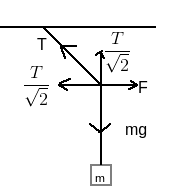Equating the vertical force

Equating the horizontal force

Q7. Cascaded Carnot engine is an arrangement in which the heat sink of one engine is a source for others. If the high temperature for one engine is T1, low temperature for other engines is T2 (Assume work done by both engine is same) Calculate the lower temperature of the first engine.

options:

a)      b)      c) 0   d)

Solution

Since the heat sink of one engine is a source for other

So

And Work done by 1st engine = work done by 2nd engine

Let the lower temperature of the first engine=T

And in Carnot engine

So

Q8. The activity of a substance changes from 700 s–1 to 500 s–1 in 30 minutes. Find its half-life in minutes

options-  a)66   b)50    c)62    d)56

Solution

Activity at any time is given as

For half-life

so

Now for

from( (i) and (ii)

Q9. In YDSE, the separation between slits is 0.15 mm, the distance between slits and screen is 1.5 m and the wavelength of light is 589 nm, then the fringe width is

Options- a)5.9 mm    b)1.9 mm   c)3.9 mm    d)2.3 mm

Solution

Given d=0.15 mm

D=1.5 m

Fringe width

The fringe width is 5.9 mm

Q10. An ideal fluid is flowing in a pipe in streamline flow. A pipe has a maximum and minimum diameter of 6.4 cm and 4.8 cm respectively. Find out the ratio of minimum to maximum velocity.

Solution

By equation of continuity

If the area is large velocity is minimum

Therefor

Q11. There is an electric circuit as shown in the figure. Find the potential difference between points a and b.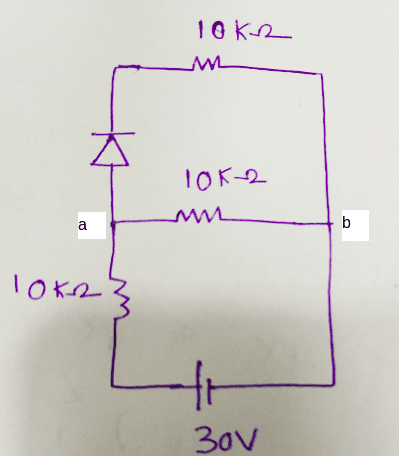Solution

A diode is forward biased

So the circuit will be as follows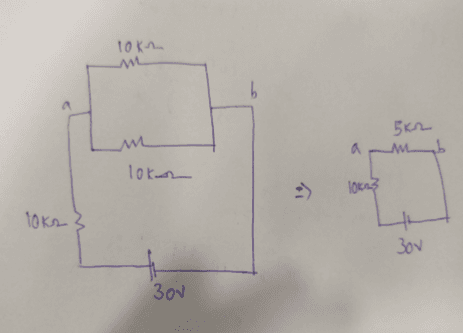The potential difference between a and b is

Q12. A particle of mass m and positive charge q is projected with a speed of v0 in the y-direction in the presence of electric and magnetic fields are in the x-direction. Find the instant of time at which the speed of particle becomes double the initial speed.

Options-

a)     b)     c)    d)

Solution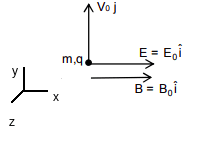Initially

As both electric and magnetic field is in the x-direction

And initial velocity is perpendicular to both electric and magnetic fields.

So particle will move in a helical motion

and magnitude of velocity does not change in y–z plane

and Speed will be increased due to electric filed only i.e in the x-direction

and finally, the speed of the particle becomes double the initial speed.

i.e

And in x-direction

So

Q13.  In an adiabatic process, the volume is doubled then find the ratio of final average relaxation time and initial relaxation time

Solution

relaxation time=

and using  gives

Q14. Two sources of sound moving with the same speed v and emitting a frequency of 1400 Hz are moving such that one source s1 is moving towards the observer and s2 is moving away from an observer. If the observer hears a beat frequency of 2 Hz. Then find the speed of the source. Given Vsound >> VSource and Vsound = 350 m/s.

Solution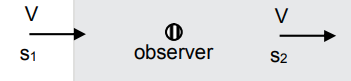given f0=1400Hz

Now

Q15.  An electron & a photon have the same energy E. Find the ratio of de Broglie wavelength of an electron to the wavelength of the photon. Given the mass of an electron is m & speed of light is c.

Solution

Q16.  A ring is rotated about a diametric axis in a uniform magnetic field perpendicular to the plane of the ring. If initially, the plane of the ring is perpendicular to the magnetic field. Find the instant of time at which EMF will be maximum & minimum respectively: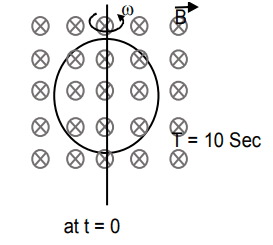Solution

We know that

And

Q17.  The electric field in space is given by . A positively charged particle at  is given velocity . The direction of force acting on a particle is:-

Solution

Force due to electric field is in direction
because at t=0,
Force due to the magnetic field is in direction
therefore, it is parallel to
therefore the net force is antiparallel to

Q18.  The focal length of the convex lens in air is . Now the lens is submerged in a liquid of refractive index 1.42. Find the ratio of focal length in medium to focal length in the air:-

Solution

Q19. The hysteresis curve for the material is shown in the figure. Then for the material retentivity, coercivity and saturation magnetization respectively will be -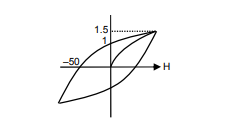(1) 50 A/m, 1T, 1.5 T   (3) 1 T, 50 A/m, 1.5 T

(2) 1.5 T, 50 A/m, 1T   (4) 50 A/m, 1.5 T, 1 T

Solution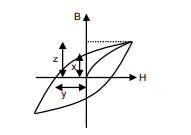So, from the figure, we can see that the-

x = retentivity, y = coercivity, z = saturation magnetization

So, by matching with the diagram from the question and solution, option 3 is correct.

Q20.  An inductor of inductance 10 mH and a resistance of 5 is connected to a battery of 20 V at t = 0. Find the ratio of current in the circuit at t =  to current at t = 40 sec.

(1) 1.06  (2) 1.48

(3) 1.15  (4) 0.84

Solution

As we know that the -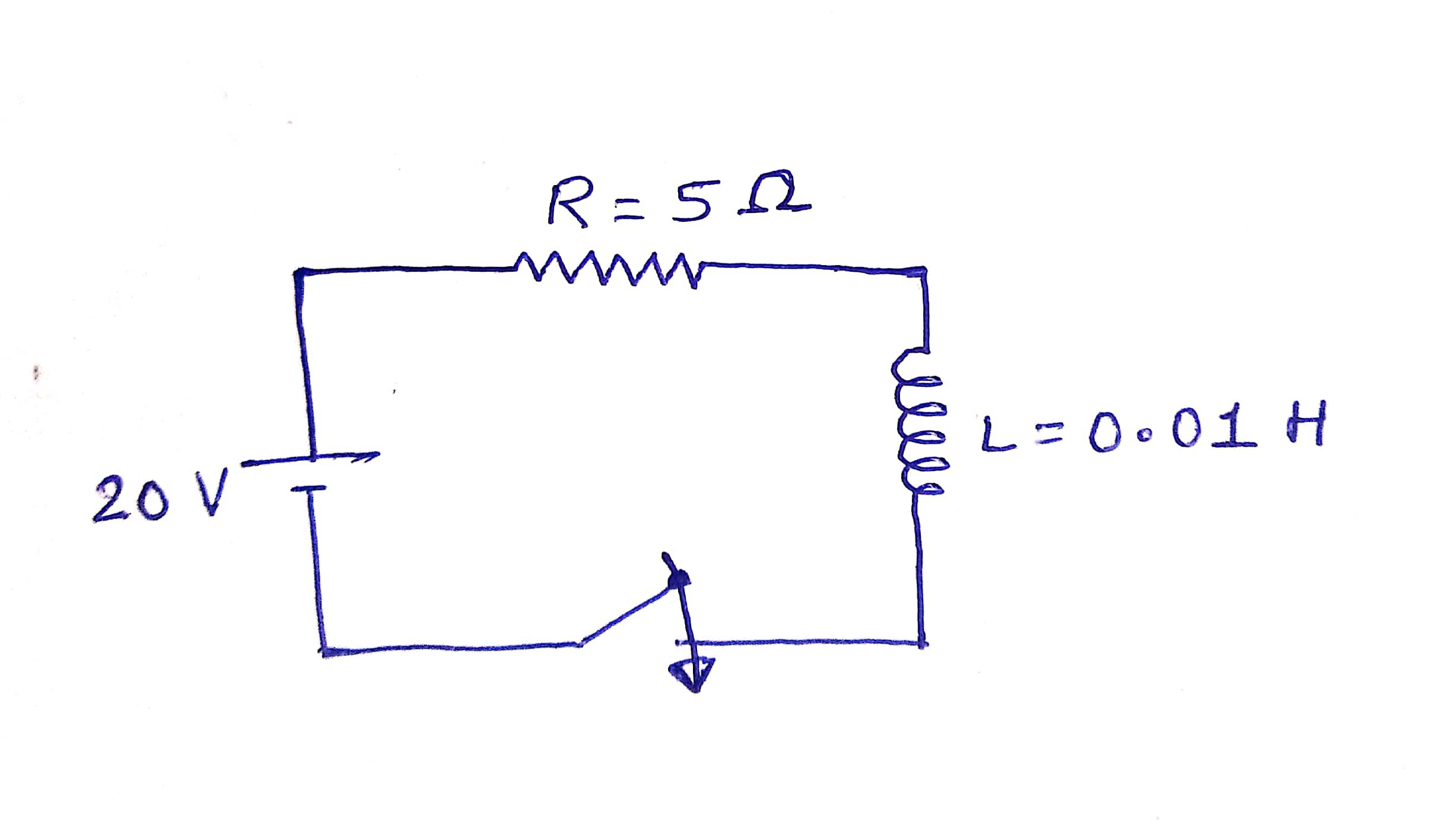So, from the question, we can write that -

By putting t =

We get,

Again at t = 40 sec

So,

So option 1 is much closer. So option 1 will be correct.

Q21.  A solid cube of side 'a' is shown in the figure. Find the maximum value of   for which the block does not topple before sliding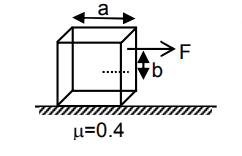solution

For no toppling

Using

using

i.e  but this is not possible as b can maximum be equal to 0.5a

So

Q22. Magnitude of resultant of two vectors   and   is equal to magnitude of . Find the angle between and resultant of    and   .

Solution

As

Now Vector P is doubled but vector Q is the same so the angle between  will be also

And If resultant of  is

Then from the figure angle between  is

and

Q23.  When m gram of steam at 100°C is mixed with 200 gm of ice at 0°C. it results in the water at 40°C. Find the value of m in gram. (given : Latent heat of fusion (Lf) = 80 cal/gm, Latent heat of vaporisation (Lv) = 540 cal/gm., specific heat of water (Cw)= 1 cal/gm/°C)

Solution

Q24.  A battery of unknown emf connected to a potentiometer has to balance length of 560 cm. If a resistor of resistance 10 ohm is connected in parallel with the cell the balancing length change by 60 cm. If the internal resistance of the cell is n/10 ohm, the value of 'n' is

Q25.  A capacitor of 60 pF charged to 20 volt. Now battery is removed and then this capacitor is connected to another identical uncharged capacitor. Find heat loss in nJ

solution

Therefor n =6

Stay tuned with us for further updates.